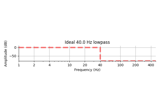# mne.filter.construct_iir_filter¶

mne.filter.construct_iir_filter(iir_params, f_pass=None, f_stop=None, sfreq=None, btype=None, return_copy=True, verbose=None)[source]

Use IIR parameters to get filtering coefficients.

This function works like a wrapper for iirdesign and iirfilter in scipy.signal to make filter coefficients for IIR filtering. It also estimates the number of padding samples based on the filter ringing. It creates a new iir_params dict (or updates the one passed to the function) with the filter coefficients (‘b’ and ‘a’) and an estimate of the padding necessary (‘padlen’) so IIR filtering can be performed.

Parameters
iir_params`dict`

Dictionary of parameters to use for IIR filtering.

• If `iir_params['sos']` exists, it will be used as second-order sections to perform IIR filtering.

New in version 0.13.

• Otherwise, if `iir_params['b']` and `iir_params['a']` exist, these will be used as coefficients to perform IIR filtering.

• Otherwise, if `iir_params['order']` and `iir_params['ftype']` exist, these will be used with `scipy.signal.iirfilter` to make a filter. You should also supply `iir_params['rs']` and `iir_params['rp']` if using elliptic or Chebychev filters.

• Otherwise, if `iir_params['gpass']` and `iir_params['gstop']` exist, these will be used with `scipy.signal.iirdesign` to design a filter.

• `iir_params['padlen']` defines the number of samples to pad (and an estimate will be calculated if it is not given). See Notes for more details.

• `iir_params['output']` defines the system output kind when designing filters, either “sos” or “ba”. For 0.13 the default is ‘ba’ but will change to ‘sos’ in 0.14.

f_pass

Frequency for the pass-band. Low-pass and high-pass filters should be a float, band-pass should be a 2-element list of float.

f_stop

Stop-band frequency (same size as f_pass). Not used if ‘order’ is specified in iir_params.

sfreq

The sample rate.

btype`str`

Type of filter. Should be ‘lowpass’, ‘highpass’, or ‘bandpass’ (or analogous string representations known to `scipy.signal.iirfilter()`).

return_copybool

If False, the ‘sos’, ‘b’, ‘a’, and ‘padlen’ entries in `iir_params` will be set inplace (if they weren’t already). Otherwise, a new `iir_params` instance will be created and returned with these entries.

verbose

If not None, override default verbose level (see `mne.verbose()` and Logging documentation for more). If used, it should be passed as a keyword-argument only.

Returns
iir_params`dict`

Updated iir_params dict, with the entries (set only if they didn’t exist before) for ‘sos’ (or ‘b’, ‘a’), and ‘padlen’ for IIR filtering.

Notes

This function triages calls to `scipy.signal.iirfilter()` and `scipy.signal.iirdesign()` based on the input arguments (see linked functions for more details).

Changed in version 0.14: Second-order sections are used in filter design by default (replacing `output='ba'` by `output='sos'`) to help ensure filter stability and reduce numerical error.

Examples

iir_params can have several forms. Consider constructing a low-pass filter at 40 Hz with 1000 Hz sampling rate.

In the most basic (2-parameter) form of iir_params, the order of the filter ‘N’ and the type of filtering ‘ftype’ are specified. To get coefficients for a 4th-order Butterworth filter, this would be:

```>>> iir_params = dict(order=4, ftype='butter', output='sos')
>>> iir_params = construct_iir_filter(iir_params, 40, None, 1000, 'low', return_copy=False)
>>> print((2 * len(iir_params['sos']), iir_params['padlen']))
(4, 82)
```

Filters can also be constructed using filter design methods. To get a 40 Hz Chebyshev type 1 lowpass with specific gain characteristics in the pass and stop bands (assuming the desired stop band is at 45 Hz), this would be a filter with much longer ringing:

```>>> iir_params = dict(ftype='cheby1', gpass=3, gstop=20, output='sos')
>>> iir_params = construct_iir_filter(iir_params, 40, 50, 1000, 'low')
>>> print((2 * len(iir_params['sos']), iir_params['padlen']))
(6, 439)
```

Padding and/or filter coefficients can also be manually specified. For a 10-sample moving window with no padding during filtering, for example, one can just do:

```>>> iir_params = dict(b=np.ones((10)), a=[1, 0], padlen=0)
>>> iir_params = construct_iir_filter(iir_params, return_copy=False)
>>> print((iir_params['b'], iir_params['a'], iir_params['padlen']))
(array([1., 1., 1., 1., 1., 1., 1., 1., 1., 1.]), [1, 0], 0)
```

For more information, see the tutorials Background information on filtering and Filtering and resampling data.

## Examples using `mne.filter.construct_iir_filter`¶